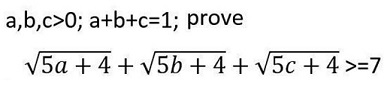# An Inequality with Constraint XI

### Problem### Solution 1

Since function $y=\sqrt{x}\;$ is concave for $x\ge 0,\;$ then according to Jensen's inequality, for $\alpha\in (0,1)\;$ and $u,v\ge 0,$

$\sqrt{\alpha u + (1-\alpha) v}\ge \alpha\sqrt{u}+(1-\alpha )\sqrt{v}.$

If so, $\sqrt{5a+4}=\sqrt{a\cdot 9+(1-a)\cdot 4}\ge a\sqrt{9}+(1-a)\sqrt{4}=a+2.\;$ Similarly, $\sqrt{5b+4}\ge b+2,\;$ $\sqrt{5c+4}\ge c+2.\;$ Adding up, gives

\displaystyle\begin{align} \sqrt{5a+4}+\sqrt{5b+4}+\sqrt{5c+4}&\ge (a+2)+(b+2)+(c+2)\\ &=(a+b+c)+6\\ &= 7. \end{align}

### Solution 2

To start with, $f(x)\ge x+2$:### Solution 3

$\sqrt{5x+4}\ge x+2,\;$ for, upon squaring, it is seen to be equivalent to $0\ge a(a-1)\;$ which is true. Now add up.

### Solution 4

$\sqrt{5b+4}+\sqrt{5c+4}\ge\sqrt{5(b+c)+4}+2=\sqrt{9-5a}+2.\,$ Therefore,

$\sqrt{5a+4}+\sqrt{5b+4}+\sqrt{5c+4}\ge\sqrt{5a+4}+\sqrt{9-5a}+2.$

Hence, suffice it to show that $\sqrt{5a+4}+\sqrt{9-5a}\ge 5,\;$ for $a\in [0,1],\;$ which is obvious.

### Solution 5### Acknowledgment

Marian Dinca has has kindly shared a problem by Lê Vãn Hai which was posted by Leo Giugiuc at the Mathematical Inequalities facebook group. Solution 1 above is by Marian Dinca. Later Leo Giugiuc pointed to more solutions. Solution 2 is by Daniel Dan; Solution 3 by Imad Zak and, independently, by Ayad Reda; Solution 4 by Leo Giugiuc; Solution 5 is by Nassim Nicholas Taleb.

The inequality is a specific case of a general statement by Dorin Marghidanu

### Inequalities with the Sum of Variables as a Constraint